• 算法时间复杂度中，我们块了解了算法时间复杂度相关的内容，比如常见的时间复杂度、时间复杂度的计算、以及提供了几简单的例子来推算时间复杂度。今天就来了解下算法空间复杂度。今天的文章很短，很容易懂~
在 算法时间复杂度 中，我们一块了解了算法时间复杂度相关的内容，比如常见的时间复杂度、时间复杂度的计算、以及提供了几个简单的例子来推算时间复杂度。今天就一块来了解下算法的空间复杂度。
首先说下空间，拿吃饭举例子吧：咱们把做好的菜从锅里吃到肚里是需要碗的吧（你要是就着锅吃饭那就……），如果锅里的菜很多你还想一次性弄出来，是不是有两种方案。一种是找个尽可能大的碗，一种是找很多个碗。算法也是一样，数据从输入到输出中间的处理过程是需要容器的，换句话说就是需要空间的。
在上篇介绍时间复杂度的时候，我们知道了说一个算法的时间复杂度并不是说一个算法的具体的执行时间的。空间复杂度也同理，并不是说一个算法执行需要的具体空间大小。
定义
说下具体的定义吧：算法的空间复杂度通过计算算法所需的存储空间实现，算法空间复杂度的计算公式记作：S(n)=O(f(n))，其中，n为问题的规模，f(n)为语句关于n所占存储空间的函数。
上段话中有个重点需要说下，和我举的例子也是有关系的：所需的存储空间指的是一个算法在运行过程中的临时占用存储空间，就像我举例中的碗一样，只是临时用的。

一个算法在计算机存储器上所占用的存储空间，包括存储算法本身所占用的存储空间，算法的输入输出数据所占用的存储空间和算法在运行过程中临时占用的存储空间这三个方面。

几种情况
常量空间
类似于时间复杂度 O(1)，当算法的存储空间大小固定，和输入规模没有直接的关系时，空间复杂度记作O(1)。
int m = 2;

线性空间
当算法分配的空间是一个线性的集合（如数组），并且集合大小和输入规模 n 成正比时，空间复杂度记作 O(n)。
int[] array = new int[n]

二维空间
当算法分配的空间是一个二维数组集合，并且集合的长度和宽度都与输入规模 n 成正比时，空间复杂度记作 O($n^2$)。
int[][] matrix = new int[n][n];


类似时间复杂度，如果为 int[][] matrix = new int[m][n]; 的话，复杂度就是 O($mn$) 了。

递归空间
先说下递归吧，说白了就是自己调用自己，比如下面的代码：
void fun4(int n){
if(n<=1){
return;
}
fun4(n-1);
…
}

再说下递归空间吧，正如上述代码一样，递归代码中没有显示声明变量或者集合，但是计算机在执行程序时，会专门分配一块内存，用来存储“方法调用栈”。方法调用栈包括入栈和出栈两个操作：

当进入一个新方法时，执行入栈操作，把调用的方法和参数信息压入栈中。
当方法返回时，执行出栈操作，把调用的方法和参数信息从栈中弹出。

还是上述代码，假设现在传入参数 5，那么方法 fun4(5) 的调用信息先入栈：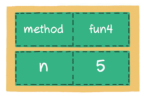接下来递归调用相同的方法，方法 fun4(4) 的调用信息入栈：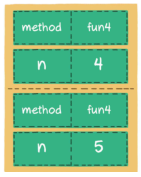依次类推，递归越来越深，栈内的元素也越来越多，最终形成下图：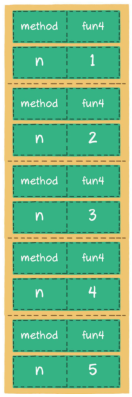当 n=1 的时候，触发递归的结束条件，执行 return，方法出栈。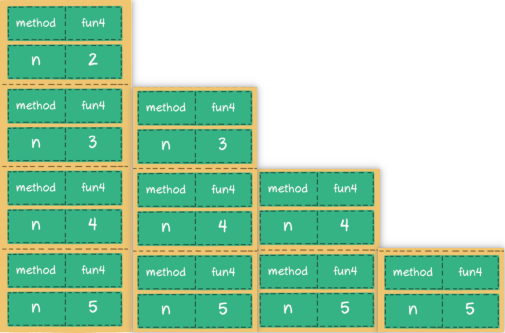最终，所有入栈的元素都会出栈。
由上面“方法调用栈”的出入栈过程可以看出，执行递归操作所需要的内存空间和递归的深度成正比。纯粹的递归操作的空间复杂度也是线性的，如果递归的深度是n，那么空间复杂度就是 O(n)。

此部分的栈的相关特性以后会有博文介绍，请期待~

两种思想
时间换空间与空间换时间

TODO：等看力扣的时候补充具体的案例


展开全文数据结构
• 算法复杂度分为时间复杂度和空间复杂度。 时间复杂度用于度量算法执行的时间长短；而空间复杂度则是用于度量算法所需存储空间的大小。 目录 时间复杂度 1.时间频度 2.计算方法 3.分类 空间复杂度 算法的时间...
文章地址：http://lzw.me/a/algorithm-complexity.html

算法复杂度分为时间复杂度和空间复杂度。
时间复杂度用于度量算法执行的时间长短；而空间复杂度则是用于度量算法所需存储空间的大小。

目录

时间复杂度

1.时间频度

2.计算方法

3.分类

空间复杂度

算法的时间复杂度（计算实例）

算法复杂度的渐近表示法

一  大O记号

二  Ω记号

三  Θ记号

四  小o记号

五  例子

常见排序算法时空复杂度

时间复杂度

1.时间频度

一个算法执行所耗费的时间，从理论上是不能算出来的，必须上机运行测试才能知道。但我们不可能也没有必要对每个算法都上机测试，只需知道哪个算法花费的时间多，哪个算法花费的时间少就可以了。并且一个算法花费的时间与算法中语句的执行次数成正比例，哪个算法中语句执行次数多，它花费时间就多。一个算法中的语句执行次数称为语句频度或时间频度。记为T(n)。

2.计算方法

1. 一般情况下，算法的基本操作重复执行的次数是模块n的某一个函数f（n），因此，算法的时间复杂度记做：T（n）=O（f（n））
分析：随着模块n的增大，算法执行的时间的增长率和f（n）的增长率成正比，所以f（n）越小，算法的时间复杂度越低，算法的效率越高。

2. 在计算时间复杂度的时候，先找出算法的基本操作，然后根据相应的各语句确定它的执行次数，再找出T（n）的同数量级（它的同数量级有以下：1，Log2n ，n ，nLog2n ，n的平方，n的三次方，2的n次方，n！），找出后，f（n）=该数量级，若T(n)/f(n)求极限可得到一常数c，则时间复杂度T（n）=O（f（n））

例：算法：

for（i=1;i<=n;++i）
{
for(j=1;j<=n;++j)
{
c[ i ][ j ]=0; //该步骤属于基本操作执行次数：n的平方 次
for(k=1;k<=n;++k)
c[ i ][ j ]+=a[ i ][ k ]*b[ k ][ j ]; //该步骤属于基本操作 执行次数：n的三次方 次
}
}

则有 T（n）= n的平方+n的三次方，根据上面括号里的同数量级，我们可以确定 n的三次方 为T（n）的同数量级
则有f（n）= n的三次方，然后根据T（n）/f（n）求极限可得到常数c
则该算法的 时间复杂度：T（n）=O（n的三次方）

3.分类

按数量级递增排列，常见的时间复杂度有：
常数阶O(1),对数阶O(log2n),线性阶O(n),
线性对数阶O(nlog2n),平方阶O(n2)，立方阶O(n3),…，
k次方阶O(nk), 指数阶O(2n) 。随着问题规模n的不断增大，上述时间复杂度不断增大，算法的执行效率越低。

空间复杂度

与时间复杂度类似，空间复杂度是指算法在计算机内执行时所需存储空间的度量。记作:
S(n)=O(f(n))
我们一般所讨论的是除正常占用内存开销外的辅助存储单元规模。

算法的时间复杂度（计算实例）

算法的时间复杂度
定义：如果一个问题的规模是n，解这一问题的某一算法所需要的时间为T(n)，它是n的某一函数 T(n)称为这一算法的“时间复杂性”。

当输入量n逐渐加大时，时间复杂性的极限情形称为算法的“渐近时间复杂性”。

我们常用大O表示法表示时间复杂性，注意它是某一个算法的时间复杂性。大O表示只是说有上界，由定义如果f(n)=O(n)，那显然成立f(n)=O(n^2)，它给你一个上界，但并不是上确界，但人们在表示的时候一般都习惯表示前者。

此外，一个问题本身也有它的复杂性，如果某个算法的复杂性到达了这个问题复杂性的下界，那就称这样的算法是最佳算法。

“大O记法”：在这种描述中使用的基本参数是 n，即问题实例的规模，把复杂性或运行时间表达为n的函数。这里的“O”表示量级 (order)，比如说“二分检索是 O(logn)的”,也就是说它需要“通过logn量级的步骤去检索一个规模为n的数组”记法 O ( f(n) )表示当 n增大时，运行时间至多将以正比于 f(n)的速度增长。

这种渐进估计对算法的理论分析和大致比较是非常有价值的，但在实践中细节也可能造成差异。例如，一个低附加代价的O(n2)算法在n较小的情况下可能比一个高附加代价的 O(nlogn)算法运行得更快。当然，随着n足够大以后，具有较慢上升函数的算法必然工作得更快。

O(1)

Temp=i;i=j;j=temp;

以上三条单个语句的频度均为1，该程序段的执行时间是一个与问题规模n无关的常数。算法的时间复杂度为常数阶，记作T(n)=O(1)。如果算法的执行时间不随着问题规模n的增加而增长，即使算法中有上千条语句，其执行时间也不过是一个较大的常数。此类算法的时间复杂度是O(1)。

O(n^2)

2.1. 交换i和j的内容

sum=0； （一次）
for(i=1;i<=n;i++) （n次）
for(j=1;j<=n;j++) （n^2次）
sum++； （n^2次）

解：T(n)=2n^2+n+1 =O(n^2)

2.2.

for (i=1;i<n;i++)
{
y=y+1; ①
for (j=0;j<=(2*n);j++)
x++; ②
}

解：语句1的频度是n-1
语句2的频度是(n-1)*(2n+1)=2n^2-n-1
f(n)=2n^2-n-1+(n-1)=2n^2-2
该程序的时间复杂度T(n)=O(n^2).

O(n)

2.3.
a=0;
b=1; ①
for (i=1;i<=n;i++) ②
{
s=a+b;　　　　③
b=a;　　　　　④
a=s;　　　　　⑤
}

解：语句1的频度：2,
语句2的频度： n,
语句3的频度： n-1,
语句4的频度：n-1,
语句5的频度：n-1,
T(n)=2+n+3(n-1)=4n-1=O(n).

O(log2n )

2.4.

i=1; ①
while (i<=n)
i=i*2; ②

解： 语句1的频度是1,
设语句2的频度是f(n), 则：2^f(n)<=n;f(n)<=log2n
取最大值f(n)= log2n,
T(n)=O(log2n )

O(n^3)

2.5.

for(i=0;i<n;i++)
{
for(j=0;j<i;j++)
{
for(k=0;k<j;k++)
x=x+2;
}
}

解：当i=m, j=k的时候,内层循环的次数为k当i=m时, j 可以取 0,1,…,m-1 , 所以这里最内循环共进行了0+1+…+m-1=(m-1)m/2次所以,i从0取到n, 则循环共进行了: 0+(1-1)*1/2+…+(n-1)n/2=n(n+1)(n-1)/6所以时间复杂度为O(n^3).

我们还应该区分算法的最坏情况的行为和期望行为。如快速排序的最坏情况运行时间是 O(n^2)，但期望时间是 O(nlogn)。通过每次都仔细地选择基准值，我们有可能把平方情况 (即O(n^2)情况)的概率减小到几乎等于 0。在实际中，精心实现的快速排序一般都能以 (O(nlogn)时间运行。

下面是一些常用的记法：

访问数组中的元素是常数时间操作，或说O(1)操作。一个算法如 果能在每个步骤去掉一半数据元素，如二分检索，通常它就取 O(logn)时间。用strcmp比较两个具有n个字符的串需要O(n)时间。常规的矩阵乘算法是O(n^3)，因为算出每个元素都需要将n对 元素相乘并加到一起，所有元素的个数是n^2。

指数时间算法通常来源于需要求出所有可能结果。例如，n个元 素的集合共有2n个子集,所以要求出所有子集的算法将是O(2n)的。指数算法一般说来是太复杂了，除非n的值非常小，因为，在 这个问题中增加一个元素就导致运行时间加倍。不幸的是，确实有许多问题 (如著名的“巡回售货员问题” )，到目前为止找到的算法都是指数的。如果我们真的遇到这种情况，通常应该用寻找近似最佳结果的算法替代之。

算法复杂度的渐近表示法

一个算法的时间复杂度，指算法运行的时间。

假设数据输入规模是n，算法的复杂度可以表示为f(n)的函数

一  大O记号

假设f(n)和g(n)的定义域是非负整数，存在两个正整数c和n0，使得n>n0的时候，f(n)≤c*g(n),则f(n)=O(g(n))。可见O(g(n))可以表示算法运行时间的上界。O(g(n))表示的函数集合的函数是阶数不超过g(n)的函数。

例如：f(n)=2*n+2=O(n)

证明：当n>3的时候，2*n +2<3n，所以可选n0=3,c=3，则n>n0的时候，f(n)<c*(n)，所以f(n)=O(n)。

现在再证明f(n)=2*n+2=O(n^2)

证明：当n>2的时候，2*n+2<2*n^2，所以可选n0=2,c=2,则n>n0的时候，f(n)<c*(n^2)，所以f(n)=O(n^2)。

同理可证f(n)=O(n^a)，a>1

二  Ω记号

Ω记号与大O记号相反，他可以表示算法运行时间的下界。Ω（g（n））表示的函数集合的函数是所有阶数超过g(n)的函数。

例如：f(n)=2*n^2+3*n+2=Ω(n^2)

证明：当n>4的时候，2*n^2+3*n+2>n^2，所以可选n0=4,c=1,则n>n0的时候，f(n)>c*(n^2)，所以f(n)=Ω(n^2)。

同理可证f(n)=Ω(n),f(n)=Ω(1)

三  Θ记号

Θ记号介于大O记号和Ω记号之间。他表示，存在正常数c1,c2,n0，当n>n0的时候，c1*g(n)≤f(n)≤c2*g(n)，则f(n)=Θ(g(n))。他表示所有阶数与g(n)相同的函数集合。

四  小o记号

f(n)=o(g(n))当且仅当f(n)=O(g(n))且f(n)≠Ω(g(n))。也就是说小o记号可以表示时间复杂度的上界，但是一定不等于下界。

五  例子

假设f(n)=2n^2+3n+5，

则f(n)=O(n^2)或者f(n) = O(n^3)或者f(n)=O(n^4)或者……

f(n)=Ω(n^2)或者f(n)=Ω(n)或者f(n)=Ω(1)

f(n)=Θ(n^2)

f(n) = o(n^3)或者f(n)=o(n^4)或者f(n)=o(n^5)或者……

注：n^2表示n的平方，以此类推。

常见排序算法时空复杂度

排序法

最差时间分析
平均时间复杂度
稳定度
空间复杂度
冒泡排序
O(n2)
O(n2)
稳定
O(1)
快速排序
O(n2)
O(n*log2n)
不稳定
O(log2n)~O(n)
选择排序
O(n2)
O(n2)
稳定
O(1)
二叉树排序
O(n2)
O(n*log2n)
不一顶
O(n)

插入排序

O(n2)
O(n2)
稳定
O(1)
堆排序
O(n*log2n)
O(n*log2n)
不稳定
O(1)
希尔排序
O
O
不稳定
O(1)

展开全文计算机领域 复杂度 时间复杂度
• 它是一个衡量算法优劣的重要指标，按照所需资源的又细分为时间复杂度和空间复杂度。 时间复杂度：运行算法所需的时间随着数据量变化关系，记做T（n），n为算法的规模。 空间复杂度一个算...
目录

概念

复杂度分析

时间复杂度分析

空间复杂度分析

总结

概念

算法复杂度：是指算法在编写成可执行程序后，运行时所需要的资源，包括时间资源（运行算法耗费的时间）和内存资源（程序运行占用的内存大小）。它是一个衡量算法优劣的重要指标，按照所需资源的又细分为时间复杂度和空间复杂度。

时间复杂度：运行算法所需的时间随着数据量变化关系，记做T（n），n为算法的规模。

空间复杂度：一个算法在运行过程中临时占用存储空间大小的量度，记做S（n），n为算法的规模。

大O表示法：表示算法时间和空间复杂度的符号，通常表示为记做S（n）=O（f（n）），T（n）= O（f（n）），n为数据的规模，f（n）通常是一个函数，比如f（n）=2n+1；f（n）=n^2；f（n）=logn等等。而T（n）一般也是一个函数以数学来说，算法运行时间和n的对应关系就是一个函数曲线，随着n逐渐增大到很大很大，除去影响该曲线主要走势的的一些因素，比如函数中的常量，系数，提取出核心因素次方，开方，对数，这个核心因素所表示的函数形式成为该算法的渐进时间空间复杂度或渐进时间复杂度，又简称时间复杂度和空间复杂度。

复杂度分析

数据结构和算法本身解决的是“快”和“省”的问题，即如何让代码运行得更快，更省存储空间。所以执行效率是算法一个非常重要的考量指标。那如何来衡量你编写的算法代码的执行效率呢？这里就需要进行复杂度分析。

事后统计法：一种在实际情况下不同环境运行测试代码，比较测试结果的方法。不过有一些局限性，一是测试结果依赖测试环境：硬件环境影响测试结果，比如Intel Core i9 处理器和 Intel Core i3 处理器差异，二是测试结果受数据规模影响：测试规模太小的可能无法真实反映算法的性能。

因此，就产生了一种不用具体的测试数据，可以只需要粗略的估算算法性能的方法，这就是我们今天要说的空间复杂度和时间复杂度，对应的分析方法又可以划分时间复杂度分析法，空间复杂度分析法。

时间复杂度分析

在前面我们说过，时间复杂度的大O表示法为T（n）= O（f（n）），那么问题来了，这个公式是怎么得出来的呢，下面就来分析下。

渐进时间复杂度官方定义：若存在函数 f（n），使得当n趋近于无穷大时，T（n）/ f（n）的极限值为不等于零的常数，则称 f（n）是T（n）的同数量级函数。记作 T（n）= O（f（n）），称O（f（n）） 为算法的渐进时间复杂度，简称时间复杂度。渐进时间复杂度用大写O来表示，所以也被称为大O表示法。

那么，问题来了，一般我们可以粗略计算出数据量n和计算所需时间T的函数关系式，如何根据已知的函数关系式推出时间复杂度呢，推理规则重点如下：

如果运行时间是常数量级，就用1表示时间复杂度；
只保留时间函数中的最高阶项；
如果最高阶项存在，则省去最高阶项前面的系数。

时间复杂度O（1）：一个方法里有四条语句，假设执行每一条语句的时间都是t，则时间和数据n的关系 T=4t。也就是说无论n怎么增加，运算的时间都是4t。同样的一个方法中语句数量为k的方法，T=kt，kt的值必然是一个常数，和n没有关系，像这种被称为常量级，根据推导公式第一条，运算时间是常量，则该T=kt的同量级函数为f（n）=1，则时间复杂度T(n)=O(1);

    public void eat(int n){
System.out.println("1111");
System.out.println("2222");
System.out.println("3333");
System.out.println("4444");
}

以函数图展示则如下，Y轴是时间复杂度，X轴是数据n的变化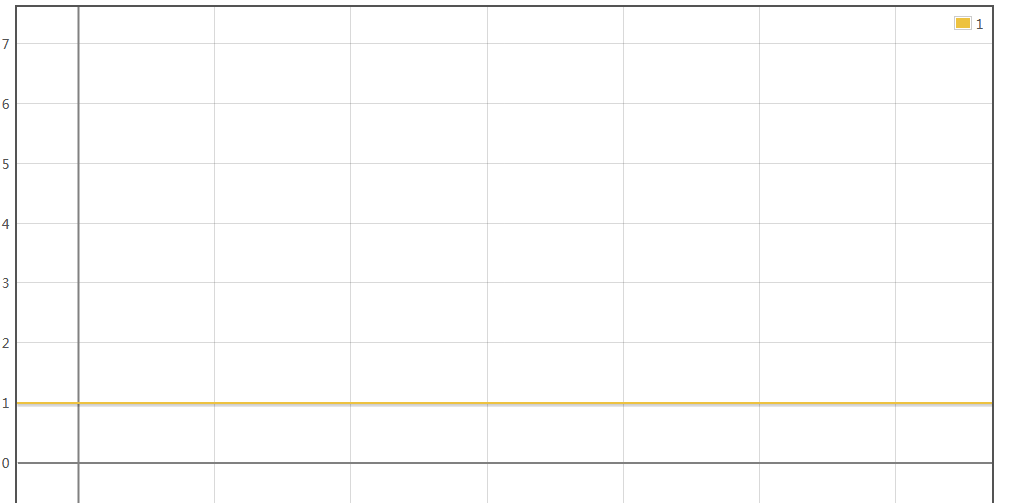时间复杂度O（n）：假设执行一条语句的时间为t，执行一次循环的时间为3t，执行n次循环的时间3tn，执行一次方法的时间为T=3tn+t，根据公式二，存在最高阶项n，所以只保留最高阶项，T=3tn；根据公式三，去除最高阶项的系数3t，则最终得到其同量级函数f（n）=n，时间复杂度公式为T（n）=O（n）；

public void eat1(int n){
System.out.println("000");
for(int i=0; i<n; i++){;
System.out.println("111");
System.out.println("222");
System.out.println("333");
}
}


以函数图展示则如下，Y轴是时间复杂度，X轴是数据n的变化，任数据变化，时间固定为常量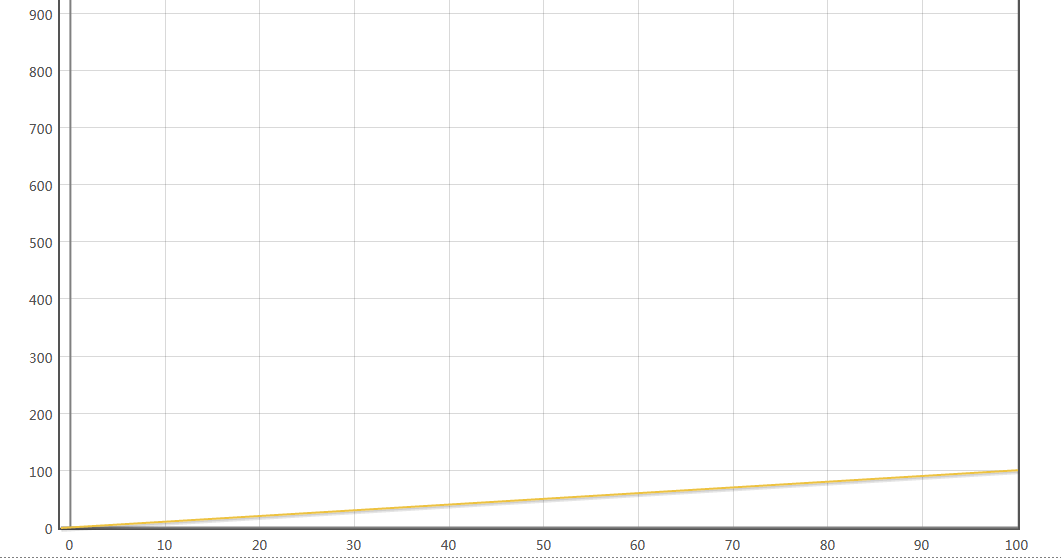时间复杂度O（n²）：假设执行一条语句的时间为t，则执行一次方法的时间T=t+n（3t+2tn），即T=t+3tn+2tn²，根据公式二出去t 3tn，根据公式三去除系数2t，最终得到同量级函数f（n）=n²；即时间复杂度T（n）=O（n²）

public void eat1(int n){
System.out.println("000");
for(int i=0; i<n; i++){;

System.out.println("111");
System.out.println("222");
System.out.println("333");

for(int k=0; k<n; k++){;
System.out.println("444");
System.out.println("555");
}
}
}

对应的函数曲线如下：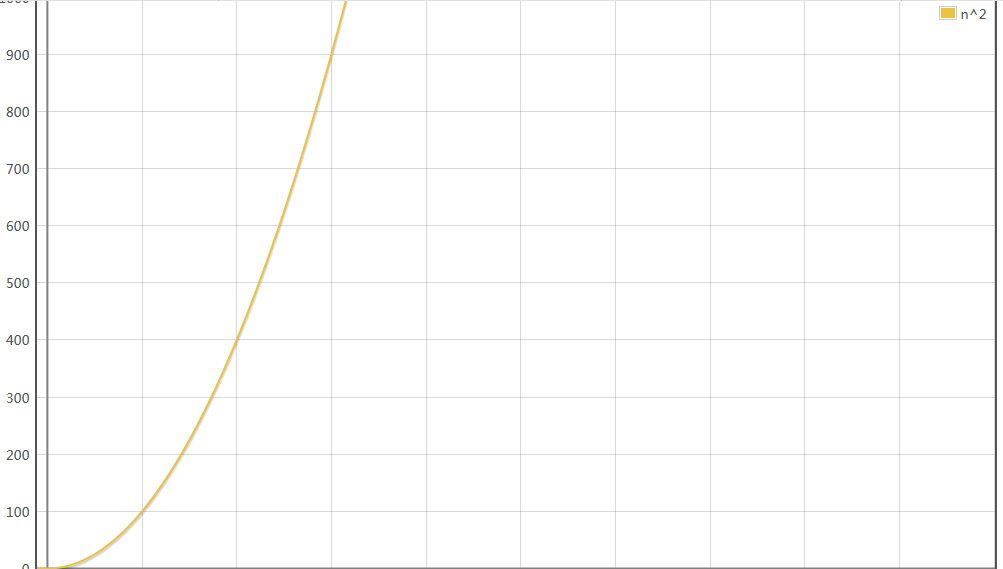同理的，其他类型的时间复杂度函数如O（logn），O（2^n），O（n³）等就不再赘述了，直接上图：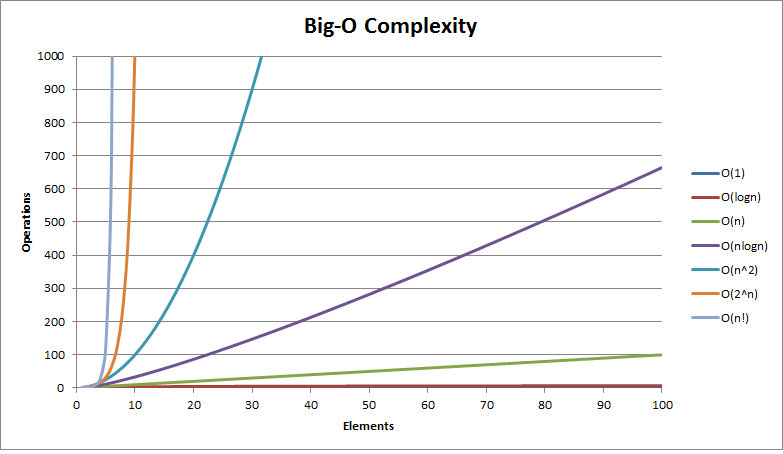空间复杂度分析

时间复杂度不是用来计算程序具体耗时的，空间复杂度也不是用来计算程序实际占用的空间的。空间复杂度是对一个算法在运行过程中临时占用存储空间大小的一个量度，同样反映的是一个趋势。空间复杂度常用的有O（1）、O（n）、O（n²），相对时间复杂度，常用的种类比较少。大多数情况下，算法的时间运行效率才是最重要的。只要算法占用的存储空间不要达到计算机无法接受的程度即可。所以，常常通过牺牲空间复杂度来换取算法更加高效的运行时间效率。

算法在计算机存储器上占用的空间包括三个部分：

输入输出：算法的输入输出数据所占用的存储空间是通过参数表由调用函数传递而来的，它不会随算法的不同而改变。

算法本身：存储算法本身所占用的存储空间与算法的长短成正比，要压缩这部分存储空间，就必须编写出较短的算法。然而，算法想要实际应用需要根据需求采取不同的编程语言来实现，不同编程语言实现的代码长短差别很大，然而存储空间都在可接受范围之内。

临时内存：临时内存是运行算法时临时占用的内存空间，分为两类。一类是占用固定内存大小，不随着问题规模变化而变化，一种是随着问题规模变化而变化。

空间复杂度O（1）：如下代码执行所需要的临时空间不随着变量n的大小而变化，即此空间复杂度为一个常量，代码中的 i、j、m 所分配的空间都不随着处理数据量变化，因此它的空间复杂度 S(n) = O(1)；

int i = 1;
int j = 2;
++i;
j++;
int m = i + j;

空间复杂度O（n）：这段代码中，第一行new了一个数组出来，这个数据占用的大小为n，这段代码的2-6行，虽然有循环，但没有再分配新的空间，因此，这段代码的空间复杂度主要看第一行即可，即 S(n) = O(n)

int[] aar = new int[n]
for(i=1; i<=n; ++i)
{
j = i;j++;
}

总结

算法的复杂度分为时间复杂度和空间复杂度，大多数情况下，更加注重时间复杂度。

通常时间复杂度近似于算法运行时间时间曲线，在数据量或者说问题规模变得很大的时候，时间复杂度高的算法耗时就高，时间复杂度低的耗时就低，从最简答到最复杂依次为：O（1）<O（logn）<O（n）<O（n²）。时间复杂度的高的通常伴随着深层次的循环和递归。减少代码的循环层次可以降低时间复杂度。

而复杂的程序代码，使用多层级的方式可以减少代码量，降低空间复杂度，层级少了较大可能需要多写代码，导致空间复杂度的提高，另外以我个人理解，对于空间的需求一般在算法初始化时就进行了预估和分配，在后续代码中虽然会产生少量临时变量，但是关键还是看初始化部分，这可能就是空间复杂度的分类比时间复杂度分类少得多。

辛辛苦苦敲了几天，拼拼凑凑加理解也算告一段落了，感谢读者的阅读，如有出入，请指正，谢谢！

展开全文时间复杂度
• 空间复杂度 是否稳定 冒泡排序 :————-: :—–: :—–: :—–: 选择排序 :————-: :—–: :—–: :—–: 直接插入排序 :————-: :—–: :—–: :—–: 归并排序 :————-: :—–: :
排序算法分类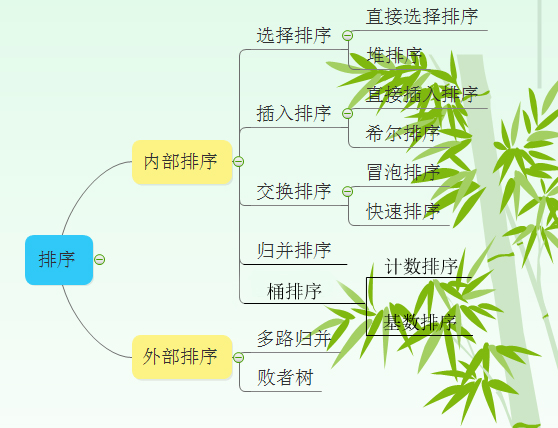排序算法比较表格填空

排序算法
平均时间复杂度
最坏时间复杂度
空间复杂度
是否稳定
冒泡排序
:————-:
:—–:
:—–:
:—–:
选择排序
:————-:
:—–:
:—–:
:—–:
直接插入排序
:————-:
:—–:
:—–:
:—–:
归并排序
:————-:
:—–:
:—–:
:—–:
快速排序
:————-:
:—–:
:—–:
:—–:
堆排序
:————-:
:—–:
:—–:
:—–:
希尔排序
:————-:
:—–:
:—–:
:—–:
计数排序
:————-:
:—–:
:—–:
:—–:
基数排序
:————-:
:—–:
:—–:
:—–:
排序算法比较表格

排序算法
平均时间复杂度
最坏时间复杂度
空间复杂度
是否稳定
冒泡排序
O（n2）<!--//--><![CDATA[//><!--
O（n^2）
//--><!]]>
O（n2）<!--//--><![CDATA[//><!--
O（n^2）
//--><!]]>
O（1）<!--//--><![CDATA[//><!--
O（1）
//--><!]]>
是
选择排序
O（n2）<!--//--><![CDATA[//><!--
O（n^2）
//--><!]]>
O（n2）<!--//--><![CDATA[//><!--
O（n^2）
//--><!]]>
O（1）<!--//--><![CDATA[//><!--
O（1）
//--><!]]>
不是
直接插入排序
O（n2）<!--//--><![CDATA[//><!--
O（n^2）
//--><!]]>
O（n2）<!--//--><![CDATA[//><!--
O（n^2）
//--><!]]>
O（1）<!--//--><![CDATA[//><!--
O（1）
//--><!]]>
是
归并排序
O(nlogn)<!--//--><![CDATA[//><!--
O(nlogn)
//--><!]]>
O(nlogn)<!--//--><![CDATA[//><!--
O(nlogn)
//--><!]]>
O（n）<!--//--><![CDATA[//><!--
O（n）
//--><!]]>
是
快速排序
O(nlogn)<!--//--><![CDATA[//><!--
O(nlogn)
//--><!]]>
O（n2）<!--//--><![CDATA[//><!--
O（n^2）
//--><!]]>
O（logn）<!--//--><![CDATA[//><!--
O（logn）
//--><!]]>
不是
堆排序
O(nlogn)<!--//--><![CDATA[//><!--
O(nlogn)
//--><!]]>
O(nlogn)<!--//--><![CDATA[//><!--
O(nlogn)
//--><!]]>
O（1）<!--//--><![CDATA[//><!--
O（1）
//--><!]]>
不是
希尔排序
O(nlogn)<!--//--><![CDATA[//><!--
O(nlogn)
//--><!]]>
O（ns）<!--//--><![CDATA[//><!--
O（n^s）
//--><!]]>
O（1）<!--//--><![CDATA[//><!--
O（1）
//--><!]]>
不是
计数排序
O(n+k)<!--//--><![CDATA[//><!--
O(n+k)
//--><!]]>
O(n+k)<!--//--><![CDATA[//><!--
O(n+k)
//--><!]]>
O(n+k)<!--//--><![CDATA[//><!--
O(n+k)
//--><!]]>
是
基数排序
O(N∗M)<!--//--><![CDATA[//><!--
O(N*M)
//--><!]]>
O(N∗M)<!--//--><![CDATA[//><!--
O(N*M)
//--><!]]>
O(M)<!--//--><![CDATA[//><!--
O(M)
//--><!]]>
是
注：

1 归并排序可以通过手摇算法将空间复杂度降到O（1），但是时间复杂度会提高。

2 基数排序时间复杂度为O（N*M），其中N为数据个数，M为数据位数。

辅助记忆

时间复杂度记忆- 冒泡、选择、直接 排序需要两个for循环，每次只关注一个元素，平均时间复杂度为O（n2）<!--//--><![CDATA[//><!--
O（n^2）
//--><!]]>（一遍找元素O(n)<!--//--><![CDATA[//><!--
O(n)
//--><!]]>，一遍找位置O(n)<!--//--><![CDATA[//><!--
O(n)
//--><!]]>）
快速、归并、希尔、堆基于二分思想，log以2为底，平均时间复杂度为O(nlogn)<!--//--><![CDATA[//><!--
O(nlogn)
//--><!]]>（一遍找元素O(n)<!--//--><![CDATA[//><!--
O(n)
//--><!]]>，一遍找位置O(logn)<!--//--><![CDATA[//><!--
O(logn)
//--><!]]>）
稳定性记忆-“快希选堆”（快牺牲稳定性） 排序算法的稳定性：排序前后相同元素的相对位置不变，则称排序算法是稳定的；否则排序算法是不稳定的。
原理理解

1 冒泡排序

1.1 过程

冒泡排序从小到大排序：一开始交换的区间为0~N-1，将第1个数和第2个数进行比较，前面大于后面，交换两个数，否则不交换。再比较第2个数和第三个数，前面大于后面，交换两个数否则不交换。依次进行，最大的数会放在数组最后的位置。然后将范围变为0~N-2，数组第二大的数会放在数组倒数第二的位置。依次进行整个交换过程，最后范围只剩一个数时数组即为有序。

1.2 动图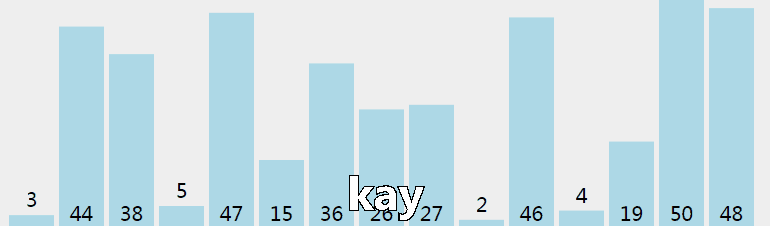1.3 核心代码（函数）

//array[]为待排序数组，n为数组长度
void BubbleSort(int array[], int n)
{
int i, j, k;
for(i=0; i<n-1; i++)
for(j=0; j<n-1-i; j++)
{
if(array[j]>array[j+1])
{
k=array[j];
array[j]=array[j+1];
array[j+1]=k;
}
}
}

2 选择排序

2.1 过程

选择排序从小到大排序：一开始从0~n-1区间上选择一个最小值，将其放在位置0上，然后在1~n-1范围上选取最小值放在位置1上。重复过程直到剩下最后一个元素，数组即为有序。

2.2 动图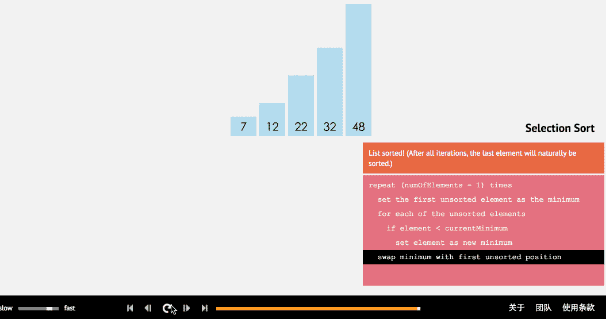2.3 核心代码（函数）

//array[]为待排序数组，n为数组长度
void selectSort(int array[], int n)
{
int i, j ,min ,k;
for( i=0; i<n-1; i++)
{
min=i; //每趟排序最小值先等于第一个数，遍历剩下的数
for( j=i+1; j<n; j++) //从i下一个数开始检查
{
if(array[min]>array[j])
{
min=j;
}
}
if(min!=i)
{
k=array[min];
array[min]=array[i];
array[i]=k;
}
}
}

3 插入排序

3.1 过程

插入排序从小到大排序：首先位置1上的数和位置0上的数进行比较，如果位置1上的数大于位置0上的数，将位置0上的数向后移一位，将1插入到0位置，否则不处理。位置k上的数和之前的数依次进行比较，如果位置K上的数更大，将之前的数向后移位，最后将位置k上的数插入不满足条件点，反之不处理。

3.2 动图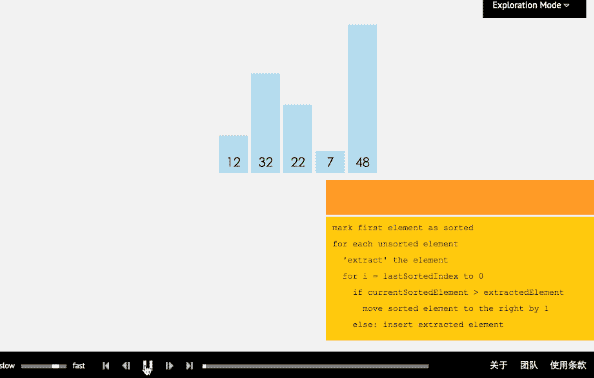3.3 核心代码（函数）

//array[]为待排序数组，n为数组长度
void insertSort(int array[], int n)
{
int i,j,temp;
for( i=1;i<n;i++)
{
if(array[i]<array[i-1])
{
temp=array[i];
for( j=i;array[j-1]>temp;j--)
{
array[j]=array[j-1];
}
array[j]=temp;
}
}
}

4 归并排序

4.1 过程

归并排序从小到大排序：首先让数组中的每一个数单独成为长度为1的区间，然后两两一组有序合并，得到长度为2的有序区间，依次进行，直到合成整个区间。

4.2 动图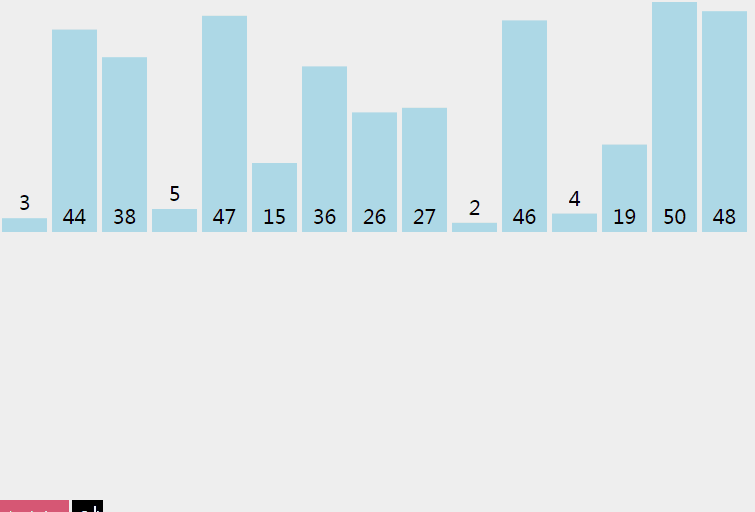4.3 核心代码（函数）

递归实现
实现归并，并把数据都放在list1里面
void merging(int *list1, int list1_size, int *list2,  int list2_size)
{
int i=0, j=0, k=0, m=0;
int temp[MAXSIZE];

while(i < list1_size && j < list2_size)
{
if(list1[i]<list2[j])
{
temp[k++] = list1[i++];
}
else
{
temp[k++] = list2[j++];
}
}
while(i<list1_size)
{
temp[k++] = list1[i++];
}
while(j<list2_size)
{
temp[k++] = list2[j++];
}

for(m=0; m < (list1_size+list2_size); m++)
{
list1[m]=temp[m];
}
}
//如果有剩下的，那么说明就是它是比前面的数组都大的，直接加入就可以了
void mergeSort(int array[], int n)
{
if(n>1)
{
int *list1 = array;
int list1_size = n/2;
int *list2 = array + n/2;
int list2_size = n-list1_size;

mergeSort(list1, list1_size);
mergeSort(list2, list2_size);

merging(list1, list1_size, list2, list2_size);
}
}
//归并排序复杂度分析：一趟归并需要将待排序列中的所有记录
//扫描一遍，因此耗费时间为O(n),而由完全二叉树的深度可知，
//整个归并排序需要进行[log2n],因此，总的时间复杂度为
//O(nlogn),而且这是归并排序算法中平均的时间性能
//空间复杂度：由于归并过程中需要与原始记录序列同样数量级的
//存储空间去存放归并结果及递归深度为log2N的栈空间，因此空间
//复杂度为O(n+logN)
//也就是说，归并排序是一种比较占内存，但却效率高且稳定的算法 

迭代实现
void MergeSort(int k[],int n)
{
int i,next,left_min,left_max,right_min,right_max;
//动态申请一个与原来数组一样大小的空间用来存储
int *temp = (int *)malloc(n * sizeof(int));
//逐级上升，第一次比较2个，第二次比较4个，第三次比较8个。。。
for(i=1; i<n; i*=2)
{
//每次都从0开始，数组的头元素开始
for(left_min=0; left_min<n-i; left_min = right_max)
{
right_min = left_max = left_min + i;
right_max = left_max + i;
//右边的下标最大值只能为n
if(right_max>n)
{
right_max = n;
}
//next是用来标志temp数组下标的，由于每次数据都有返回到K，
//故每次开始得重新置零
next = 0;
//如果左边的数据还没达到分割线且右边的数组没到达分割线，开始循环
while(left_min<left_max&&right_min<right_max)
{
if(k[left_min] < k[right_min])
{
temp[next++] = k[left_min++];
}
else
{
temp[next++] = k[right_min++];
}
}
//上面循环结束的条件有两个，如果是左边的游标尚未到达，那么需要把
//数组接回去，可能会有疑问，那如果右边的没到达呢，其实模拟一下就可以
//知道，如果右边没到达，那么说明右边的数据比较大，这时也就不用移动位置了

while(left_min < left_max)
{
//如果left_min小于left_max，说明现在左边的数据比较大
//直接把它们接到数组的min之前就行
k[--right_min] = k[--left_max];
}
while(next>0)
{
//把排好序的那部分数组返回该k
k[--right_min] = temp[--next];
}
}
}
}
//非递归的方法，避免了递归时深度为log2N的栈空间，
//空间只是用到归并临时申请的跟原来数组一样大小的空间，并且在时间性能上也有一定的提升，
//因此，使用归并排序是，尽量考虑用非递归的方法。

5 快速排序

5.1 过程

快速排序从小到大排序：在数组中随机选一个数（默认数组首个元素），数组中小于等于此数的放在左边，大于此数的放在右边，再对数组两边递归调用快速排序，重复这个过程。

5.2 动图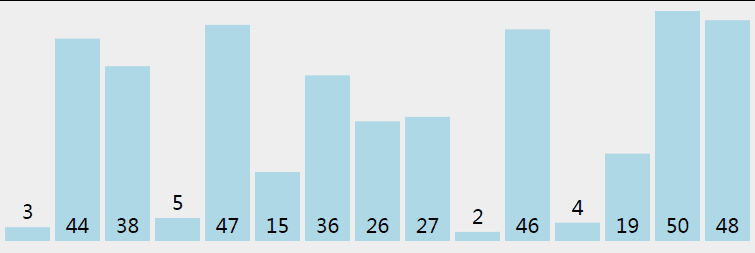5.3 核心代码（函数）

推荐程序（好理解）

//接口调整
{
quicksort(k,0,n-1);
}
void quicksort(int a[], int left, int right)
{
int i,j,t,temp;
if(left>right)   //（递归过程先写结束条件）
return;

temp=a[left]; //temp中存的就是基准数
i=left;
j=right;
while(i!=j)
{
//顺序很重要，要先从右边开始找（最后交换基准时换过去的数要保证比基准小，因为基准
//选取数组第一个数，在小数堆中）
while(a[j]>=temp && i<j)
j--;
//再找右边的
while(a[i]<=temp && i<j)
i++;
//交换两个数在数组中的位置
if(i<j)
{
t=a[i];
a[i]=a[j];
a[j]=t;
}
}
//最终将基准数归位 （之前已经temp=a[left]过了，交换只需要再进行两步）
a[left]=a[i];
a[i]=temp;

quicksort(left,i-1);//继续处理左边的，这里是一个递归的过程
quicksort(i+1,right);//继续处理右边的 ，这里是一个递归的过程
}  

6 堆排序

6.1 过程

堆排序从小到大排序：首先将数组元素建成大小为n的大顶堆，堆顶（数组第一个元素）是所有元素中的最大值，将堆顶元素和数组最后一个元素进行交换，再将除了最后一个数的n-1个元素建立成大顶堆，再将最大元素和数组倒数第二个元素进行交换，重复直至堆大小减为1。

注：完全二叉树
假设二叉树深度为n，除了第n层外，n-1层节点都有两个孩子，第n层节点连续从左到右。如下图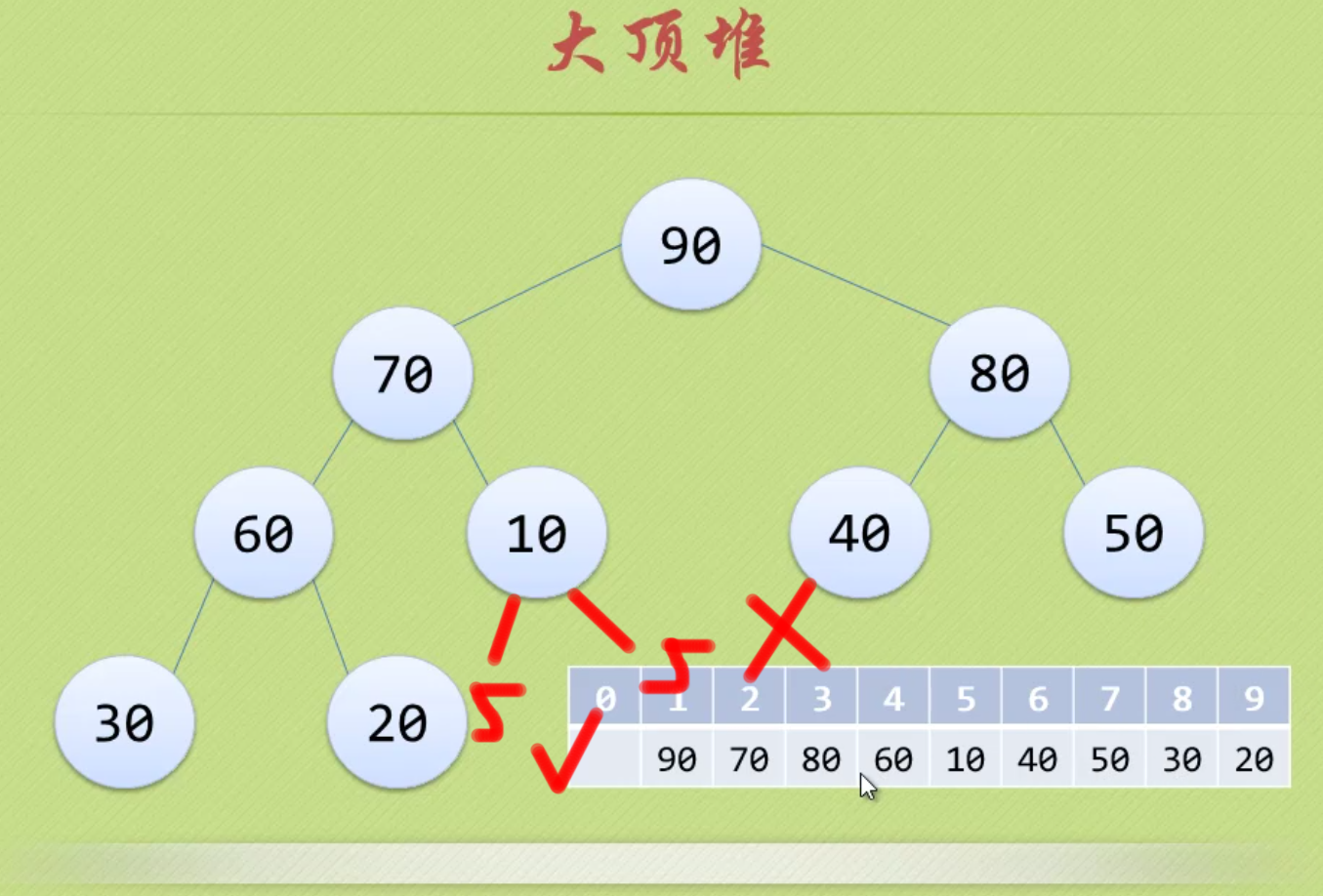注：大顶堆
大顶堆是具有以下性质的完全二叉树：每个节点的值都大于或等于其左右孩子节点的值。
即，根节点是堆中最大的值，按照层序遍历给节点从1开始编号，则节点之间满足如下关系：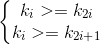(1<=i<=n/2)
6.2 动图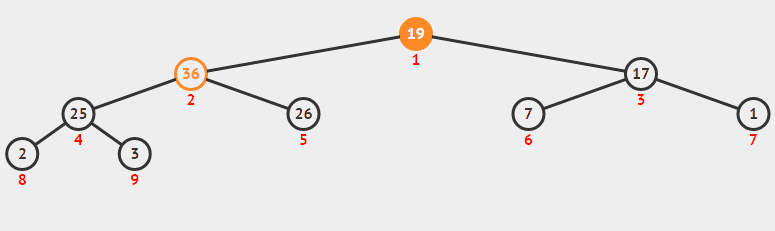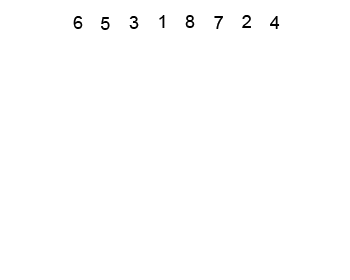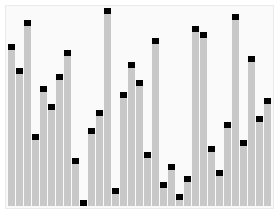6.3 核心代码（函数）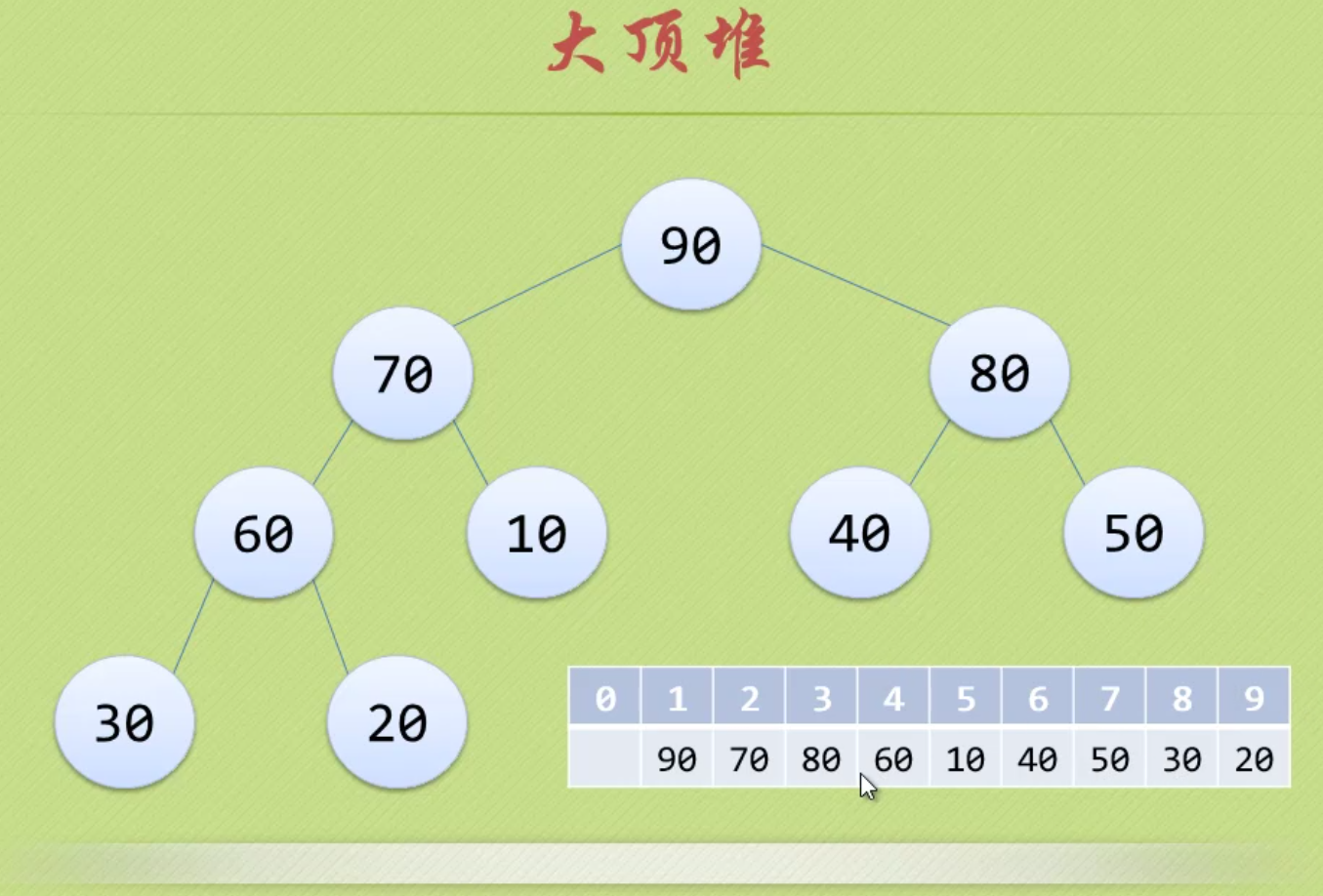注意！！！数组从1开始，1~n

void heapSort(int array[], int n)
{
int i;
for (i=n/2;i>0;i--)
{
}
for( i=n;i>1;i--)
{
swap(array, 1, i);
}
}
void HeapAdjust(int array[], int s, int n )
{
int i,temp;
temp = array[s];
for(i=2*s;i<=n;i*=2)
{
if(i<n&&array[i]<array[i+1])
{
i++;
}
if(temp>=array[i])
{
break;
}
array[s]=array[i];
s=i;
}
array[s]=temp;
}
void swap(int array[], int i, int j)
{
int temp;

temp=array[i];
array[i]=array[j];
array[j]=temp;
}

7 希尔排序

7.1 过程

希尔排序是插入排序改良的算法，希尔排序步长从大到小调整，第一次循环后面元素逐个和前面元素按间隔步长进行比较并交换，直至步长为1，步长选择是关键。

7.2 动图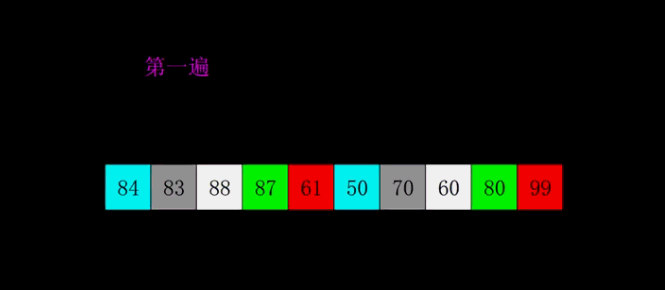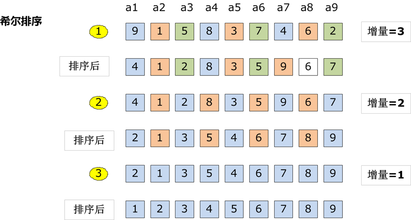7.3 核心程序（函数）

//下面是插入排序
void InsertSort( int array[], int n)
{
int i,j,temp;
for( i=0;i<n;i++ )
{
if(array[i]<array[i-1])
{
temp=array[i];
for( j=i-1;array[j]>temp;j--)
{
array[j+1]=array[j];
}
array[j+1]=temp;
}
}
}
//在插入排序基础上修改得到希尔排序
void SheelSort( int array[], int n)
{
int i,j,temp;
int gap=n; //~~~~~~~~~~~~~~~~~~~~~
do{
gap=gap/3+1;  //~~~~~~~~~~~~~~~~~~
for( i=gap;i<n;i++ )
{
if(array[i]<array[i-gap])
{
temp=array[i];
for( j=i-gap;array[j]>temp;j-=gap)
{
array[j+gap]=array[j];
}
array[j+gap]=temp;
}
}
}while(gap>1);  //~~~~~~~~~~~~~~~~~~~~~~

}

8 桶排序（基数排序和基数排序的思想）

8.1 过程

桶排序是计数排序的变种，把计数排序中相邻的m个”小桶”放到一个”大桶”中，在分完桶后，对每个桶进行排序（一般用快排），然后合并成最后的结果。

8.2 图解8.3 核心程序

#include <stdio.h>
int main()
{
int a,i,j,t;
for(i=0;i<=10;i++)
a[i]=0;  //初始化为0

for(i=1;i<=5;i++)  //循环读入5个数
{
scanf("%d",&t);  //把每一个数读到变量t中
a[t]++;  //进行计数(核心行)
}

for(i=0;i<=10;i++)  //依次判断a~a
for(j=1;j<=a[i];j++)  //出现了几次就打印几次
printf("%d ",i);

getchar();getchar();
//这里的getchar();用来暂停程序，以便查看程序输出的内容
//也可以用system("pause");等来代替
return 0;
}

9 计数排序

9.1 过程

算法的步骤如下：
- 找出待排序的数组中最大和最小的元素
- 统计数组中每个值为i的元素出现的次数，存入数组C的第i项
- 对所有的计数累加（从C中的第一个元素开始，每一项和前一项相加）
- 反向填充目标数组：将每个元素i放在新数组的第C(i)项，每放一个元素就将C(i)减去1

9.2 图解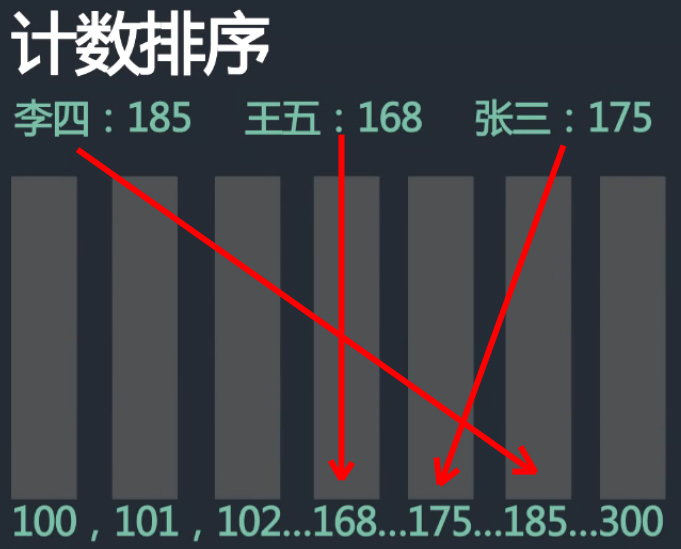9.3 核心程序（函数）

程序1：
#define NUM_RANGE (100)    //预定义数据范围上限，即K的值

void counting_sort(int *ini_arr, int *sorted_arr, int n)  //所需空间为 2*n+k
{
int *count_arr = (int *)malloc(sizeof(int) * NUM_RANGE);
int i, j, k;

//初始化统计数组元素为值为零
for(k=0; k<NUM_RANGE; k++){
count_arr[k] = 0;
}
//统计数组中，每个元素出现的次数
for(i=0; i<n; i++){
count_arr[ini_arr[i]]++;
}

//统计数组计数，每项存前N项和，这实质为排序过程
for(k=1; k<NUM_RANGE; k++){
count_arr[k] += count_arr[k-1];
}

//将计数排序结果转化为数组元素的真实排序结果
for(j=n-1 ; j>=0; j--){
int elem = ini_arr[j];          //取待排序元素
int index = count_arr[elem]-1;  //待排序元素在有序数组中的序号
sorted_arr[index] = elem;       //将待排序元素存入结果数组中
count_arr[elem]--;              //修正排序结果，其实是针对算得元素的修正
}
free(count_arr);
}

程序2：C++(最大最小压缩桶数)
public static void countSort(int[] arr) {
if (arr == null || arr.length < 2) {
return;
}
int min = arr;
int max = arr;
for (int i = 1; i < arr.length; i++) {
min = Math.min(arr[i], min);
max = Math.max(arr[i], max);
}
int[] countArr = new int[max - min + 1];
for (int i = 0; i < arr.length; i++) {
countArr[arr[i] - min]++;
}
int index = 0;
for (int i = 0; i < countArr.length; i++) {
while (countArr[i]-- > 0) {
arr[index++] = i + min;
}
}

10 基数排序

10.1 过程

基数排序是基于数据位数的一种排序算法。
它有两种算法
①LSD–Least Significant Digit first  从低位（个位）向高位排。
②MSD– Most Significant Digit first 从高位向低位（个位）排。
时间复杂度O(N*最大位数)。
空间复杂度O(N)。

10.2 图解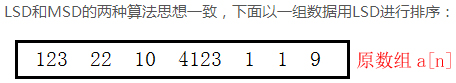对a[n]按照个位0~9进行桶排序：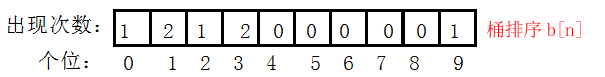对b[n]进行累加得到c[n]，用于b[n]中重复元素计数
！！！b[n]中的元素为temp中的位置！！！跳跃的用++补上：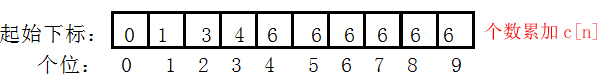temp数组为排序后的数组，写回a[n]。temp为按顺序倒出桶中的数据（联合b[n],c[n],a[n]得到），重复元素按顺序输出：10.3 核心程序

//基数排序
//LSD  先以低位排，再以高位排
//MSD  先以高位排，再以低位排
void LSDSort(int *a, int n)
{
assert(a);  //判断a是否为空，也可以a为空||n<2返回
int digit = 0;   //最大位数初始化
for (int i = 0; i < n; ++i)
{   //求最大位数
while (a[i] > (pow(10,digit)))  //pow函数要包含头文件math.h，pow(10,digit)=10^digit
{
digit++;
}
}
int flag = 1;   //位数
for (int j = 1; j <= digit; ++j)
{
//建立数组统计每个位出现数据次数（Digit[n]为桶排序b[n]）
int Digit = { 0 };
for (int i = 0; i < n; ++i)
{
Digit[(a[i] / flag)%10]++;  //flag=1时为按个位桶排序
}
//建立数组统计起始下标（BeginIndex[n]为个数累加c[n]，用于记录重复元素位置
//flag=1时，下标代表个位数值，数值代表位置，跳跃代表重复）
int BeginIndex = { 0 };
for (int i = 1; i < 10; ++i)
{
//累加个数
BeginIndex[i] = BeginIndex[i - 1] + Digit[i - 1];
}
//建立辅助空间进行排序
//下面两条可以用calloc函数实现
int *tmp = new int[n];
memset(tmp, 0, sizeof(int)*n);//初始化
//联合各数组求排序后的位置存在temp中
for (int i = 0; i < n; ++i)
{
int index = (a[i] / flag)%10;  //桶排序和位置数组中的下标
//计算temp相应位置对应a[i]中的元素，++为BeginIndex数组数值加1
//跳跃间隔用++来补，先用再++
tmp[BeginIndex[index]++] = a[i];
}
//将数据重新写回原空间
for (int i = 0; i < n; ++i)
{
a[i] = tmp[i];
}
flag = flag * 10;
delete[] tmp;
}
}  

附：

1 完整程序框架（冒泡排序举例）

1.1 VS2010程序

#include "stdafx.h"
#include "stdio.h"
#include <stdlib.h>

void BubbleSort(int array[], int n){
int i,j,k,count1=0, count2=0;
for(i=0; i<n-1; i++)
for(j=n-1; j>i; j--)
{
count1++;
if(array[j-1]>array[j])
{
count2++;
k=array[j-1];
array[j-1]=array[j];
array[j]=k;
}
}
printf("总共的循环次序为：%d,  总共的交换次序为：%d\n\n", count1, count2);
}

int main(int argc, _TCHAR* argv[])
{
int as[]={0,1,2,3,4,6,8,5,9,7};
BubbleSort(as, 10);
for(int i=0; i<10; i++)
{
printf("%d", as[i]);
}
printf("\n\n");
system("pause");
return 0;
}

1.2 执行程序（OJ）

#include <stdio.h>

void BubbleSort(int array[], int n){
int i,j,k,count1=0, count2=0;
for(i=0; i<n-1; i++)
for(j=n-1; j>i; j--)
{
count1++;
if(array[j-1]>array[j])
{
count2++;
k=array[j-1];
array[j-1]=array[j];
array[j]=k;
}
}
printf("总共的循环次序为：%d,  总共的交换次序为：%d\n\n", count1, count2);
}

int main()
{
int as[]={0,1,2,3,4,6,8,5,9,7};
BubbleSort(as, 10);
int i=0;
for(i=0; i<10; i++)
{
printf("%d", as[i]);
}
return 0;
}

2 关于交换的优化

不用中间变量进行交换

if(A[j] <= A[i]){
A[j] = A[j] + A[i];
A[i] = A[j] - A[i];
A[j] = A[j] - A[i];
}

3 C语言实现数组动态输入

#include <stdio.h>
#include <assert.h>  //断言头文件
#include <stdlib.h>

int main(int argc, char const *argv[])
{
int size = 0;
scanf("%d", &size);   //首先输入数组个数
assert(size > 0);     //判断数组个数是否非法

int *array = (int *)calloc(size, sizeof(int));  //动态分配数组
if(!R1)
{
return;           //申请空间失败
}

int i = 0;
for (i = 0; i < size; ++i) {
scanf("%d", &array[i]);
}

mergeSort(array, size);
printArray(array, size);

free(array);
return 0;
} 

注：
1.colloc与malloc类似,但是主要的区别是存储在已分配的内存空间中的值默认为0,使用malloc时,已分配的内存中可以是任意的值.
2.colloc需要两个参数,第一个是需要分配内存的变量的个数,第二个是每个变量的大小.
展开全文• 算法的时间复杂度和空间复杂度合称为算法的复杂度。 1.时间复杂度 （1）时间频度 一个算法执行所耗费的时间，从理论上是不能算出来的，必须上机运行测试才能知道。但我们不可能也没有必要对每个算法都上机测试...时间复杂度 CC++
• 空间复杂度(Space Complexity)是对一个算法在运行过程中临时占用存储空间大小的量度。 一个算法在计算机存储器上所占用的存储空间，包括程序代码所占用的空间，输入数据所占用的空间和辅助变量所占用的空间这三个...时间复杂度 斐波那契数列
•  常用的算法的时间复杂度和空间复杂度 ，求解算法的时间复杂度，其具体步骤是： 　⑴ 找出算法中的基本语句； 　算法中执行次数最多的那条语句就是基本语句，通常是最内层循环的循环体。 　⑵ 计算...java
• 算法的时间复杂度和空间复杂度 1、时间复杂度 （1）时间频度 一个算法执行所耗费的时间，从理论上是不能算出来的，必须上机运行测试才能知道。但我们不可能也没有必要对每个算法都上机测试，只需知道哪个算法花费的...时间复杂度
• (1) 复杂度 为了描述一个算法的优劣，我们引入算法时间复杂度和空间复杂度的概念。 时间复杂度：一个算法主要运算的次数，用 O 表示。通常表示时间复杂度时，我们只保留数量级最大的 项，并忽略该项的系数。 例如...
• 算法的时间复杂度一个函数，它定量描述了该算法的运行时间，时间复杂度常用“O”表述，使用这种方式时，时间复杂度可被称为是渐近的，它考察当输入值大小趋近无穷时的情况 时间复杂度是用来估计算法运行时间的...
• 1、算法的时间复杂度就是所有语句的频度之和 2、空间复杂度就是执行该算法所需要占用的内存空间 例如以下算法
• 空间复杂度 稳定性 选择排序 Selection n2 n2 n2 1 不稳 冒泡排序 Bubble ...
•  算法复杂度分为时间复杂度和空间复杂度一个好的算法应该具体执行时间短，所需空间少的特点。  随着计算机硬件和软件的提升，一个算法的执行时间是算不太精确的。只能依据统计方法对算法进行估算。我们抛开硬件...
• 算法时间复杂度的定义：在进行算法分析使，语句总的执行次数T(n)是关于问题规模n的函数，进而分析T(n)随n 的变化请款并确定T(n)的数量级。算法的时间复杂度，也就是算法的时间...我们三求和算法的时间复杂度分别为
• 各排序算法时间复杂度和空间复杂度对比总结 排序算法稳定性：是指待排序列的序列中有两或两以上相同的项，排序前和排序后，看这些相同的项的相对位置有没有发生变化。如果没有发生变化，就是稳定的；如果发生...C++ 时间复杂度 稳定性
• 评价一个算法有几标准，主要包括Correctness（正确性），Amount of work done（工作量），Amount of space used（使用空间），Simplicity（简单性），Optimality（最优性） 其中正确性涉及到数学证明；工作量表...时间复杂度
• 时间复杂度分析：XXXXXXXW：O（N^3）1秒内可以处理大约10^2量级的数据插入排序法：O（N^2）1秒内可以处理大约10^4量级的数据选择排序法：O（N^2）1秒内可以处理大约10^4量级的数据归并排序法：O（NlogN）1秒内可以...时间复杂度
• 归并排序空间复杂度为O(n) ...快速排序空间复杂度为O(logn~n):因为快速排序是递归的，需要一个栈存放相应的数据，最大递归调用次数与递归树的深度有关 堆排序空间复杂度在非递归情况下是O(1),递归情况下就是O(logn)排序 时间复杂度...